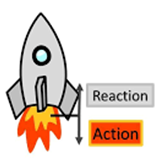Physics
Easy

Question

# Which law is being expressed in this image?## Newton’s first law of motion.Newton’s second law of motion.Newton’s third law of motion.Newton’s fourth law of motion.Hint:

## The correct answer is: Newton’s third law of motion.

### The third law states that for every action there is an equal and opposite reaction. The two bodies will experience each other's reaction forces in opposite directions. So, the correct answer is Newton's third law of motion.#### With Turito Foundation.#### Get an Expert Advice From Turito.